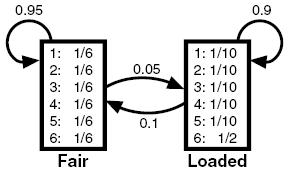First we implement the Viterbi algorithm as described in these notes: nvit.pdf
T[i,j] is the i->j transition probability, e[i][a] is the emission probability for observable a from state i, w are the initial state weights, snames is a list of the names to call the states (instead of the integers i,j), and O is a string of observations.

In :
def viterbi(T,e,w,snames,O):
states=range(len(snames))  #label states as integers
#initialize probability for first observation:
v = [w[j]*e[j][O] for j in states]
path=snames   # initialize paths to state names

for o in O[1:]:
#  nv[j]=v[i]*T[i,j] array with probabilities for arriving from i to j:
nv = [v*T[:,j] for j in states]
#  update maximum probability to state i for next step:
v = [max(nv[j])*e[j][o] for j in states]
#  path[j] keeps track of max prob path to get to state j:
path = [path[argmax(nv[j])] + snames[j] for j in states]

return path[argmax(v)],max(v)  #return path to max probability final state


First consider the simple case of three urns 1,2,3 and three observations RBR as in the above notes:

In :
T=array([[.3,.6,.1],[.5,.2,.3],[.4,.1,.5]])
e=[{'B':1./2,'R':1./2},{'B':2./3,'R':1./3},{'B':1./4,'R':3./4}]
w=ones(3)/3.
state_names=['1','2','3']
viterbi(T,e,w,state_names,'RBR')

Out:
('121', 0.016666666666666663)

In :
from IPython.display import Image
Image('casino.jpg')

Out:In :
def roll(die='F'):
if die=='F': return randint(1,7)
elif die== 'L': return random.choice([1,2,3,4,5,6,6,6,6,6])
else: return None

def trial(state):
r=rand()
if state=='F' and rand() < .05: state = 'L'
elif state=='L' and rand() < .1: state = 'F'
return state,str(roll(state))


And generate a series of 300 hidden state transitions and observations starting from the 'F' state:

In :
q='F'
states=q
rolls=str(roll(q))
for i in xrange(299):
q,o=trial(q)  #update state q, and get observed o
states += q
rolls += o

In :
for i in 0,1,2:
print rolls[100*i:100*(i+1)]
print states[100*i:100*(i+1)],'\n'

4242515462351161555223451263544366336116515561154145424646262651632521164555362346662536566466643511
FFFFFFFFFFFFFLLLFFFFFFFFFFFFFFFFFFFFFFFFFFFFFFFFFFFFFFFLLLLLLLLLLLLLLLLLLFFFLLLLLLLFLLLLLLLLLLFFFFFF

4556213455631362425354166562666661626416623151424364234166226656653231266666621665546336665546622165
FFFFFFFFFFFFFFFFFFFFFFFLLLLLLLLLLLLLLLLLLFFFFFFFFFLLFFLLLLLLLLLLLLLLLLLLLLLLLLLLLLFLLLLLLLLLLLLLLLLL

2641313326461151232644165264466616655623346562552425232663226433134456133666541325651625451255323413
LLFFFFFFFFFFFFFFFFFFFFFFFFFFLLLLFFFFFLLLFFFFFFFFFFFFFFFFFFFFFFFFLLLLLLFFFFFFFFFFFFFFFFFFFFFFFFFFFFFF



In the above the hidden states are known, but suppose we try to infer the state transitions knowing only the obervations in the middle line above:

In :
O='4556213455631362425354166562666661626416623151424364234166226656653231266666621665546336665546622165'
Q='FFFFFFFFFFFFFFFFFFFFFFFLLLLLLLLLLLLLLLLLLFFFFFFFFFLLFFLLLLLLLLLLLLLLLLLLLLLLLLLLLLFLLLLLLLLLLLLLLLLL'

T=array([[.95,.05],[.1,.9]])
snames=['F','L']

e = [{str(o): 1/6. for o in range(1,7)},
{str(o): 1/10. if o<6 else 1/2. for o in range(1,7) }]

w=[.5,.5]

print 'observed:',O
print '  hidden:',Q,'\n'
print 'inferred:',viterbi(T,e,w,snames,O)

observed: 4556213455631362425354166562666661626416623151424364234166226656653231266666621665546336665546622165
hidden: FFFFFFFFFFFFFFFFFFFFFFFLLLLLLLLLLLLLLLLLLFFFFFFFFFLLFFLLLLLLLLLLLLLLLLLLLLLLLLLLLLFLLLLLLLLLLLLLLLLL

inferred: FFFFFFFFFFFFFFFFFFFFFFFLLLLLLLLLLLLLLLLLLFFFFFFFFFFFFFFFLLLLLLLLLLLLLLLLLLLLLLLLLLLLLLLLLLLLLLLLLLLL


We see that it gets the essential large-scale features of the state transitions.

In [ ]: